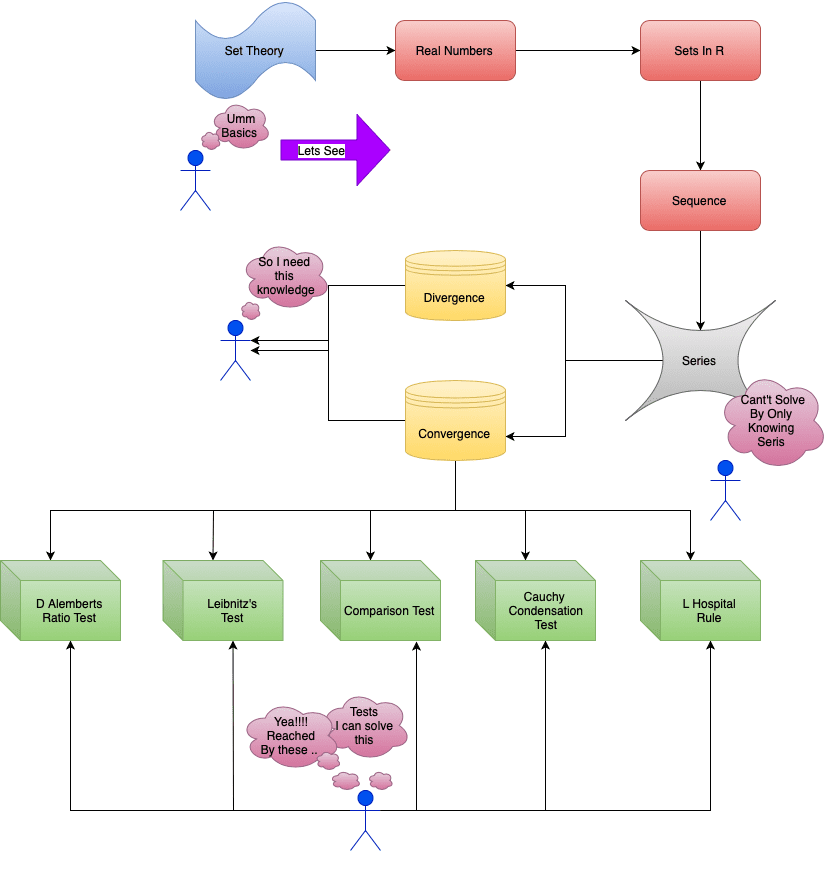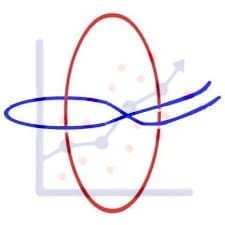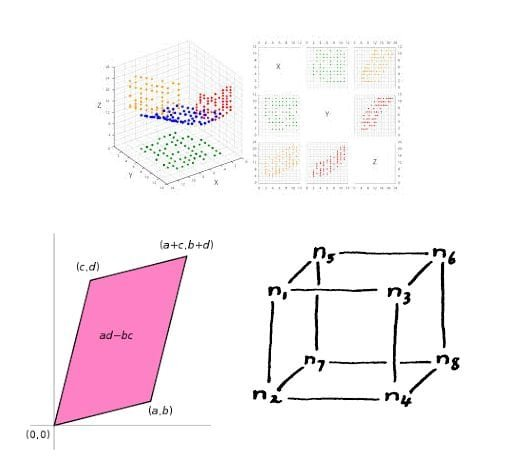Categories
College Mathematics

# Series Convergence: IIT JAM 2018 Problem 12

Well this problem appeared in IIT JAM 2018 paper . This problem is very basic one and requires basic knowledge form convergence of a seris.

[h5p id="13"]

# Understand the problem

Let a, b, c$\in \mathbb{R}$ Which of the following values of a ,b, c do NOT result in the convergence of the series$\sum_{n=3}^{\infty} \frac{a^{n}}{n^{b} (log_en)^{c}}$(a)$|a|<1 , b \in \mathbb{R} , c \in \mathbb{R}$(b)$a=1 , b>1 , c \in \mathbb{R}$(c)$a=1 , b \leq 1 , c<1$(d)$a=-1 , b \geq , c>0$
##### Source of the problem
IIT JAM 2018 Problem 12
##### Topic
Convergence of a seris
Easy
##### Suggested Book
Real Analysis By S.K Mapa

Do you really need a hint? Try it first!
One disclaimer: In this question you will  see that for some option the series is clearly convergent and for some option it might be convergent and might not be. So the question wordings are not very clear. Now having that disclaimer, what we have to find is the options where we have the series might be or might not be convergent. I want to end this hint here to  give you a bit more room to search. Look for Leibnitz rule for alternating series.[ In mathematics Leibnitz’s test states that if${u_n}$be a monotone decreasing sequence of positive real numbers and lim$u_n = 0$, then the alternating series$u_1 - u_2 + u_3 - u_4 + ...........$is convergent.]$\sum_{n=3}^{\infty} \frac{a^{n}}{n^{b} (log_e^{n})^{c}}$Let us talk about option D first$a=-1, b \geq 0 , c<0$$\sum_{n=3}^{\infty} \frac{(-1)^{n}}{n^{b}(ln^{n})^{c}}$  where$\frac{1}{n^{b}(ln n)^{c}} \longrightarrow 0$as$n \longrightarrow \infty$Hence the series is convergence in this case. So option D is rejected. Now look for the other option and see Cauchy condensation test. (For a non increasing sequence$f(n)$of non-negative real numbers, the series$\sum_{n=1}^{\infty} f(n)$converges if and only if the “condensed series”$\sum_{n=0}^{\infty} 2^{n} f(2^{n})$converges.Moreover if they converge,the sum of the condensed series is no more than twice as large of the sum as original) and D’ Alembert’s test( Let$\sum u_n$be a series of positive real numbers and let$lim \frac{u_n +1}{u_n} = l$Then$\sum u_n$is convegent if$l<1$,$\sum u_n$is divergent if$l>1$.
Moving on to option c$a=1 , b \geq 0 , c<1$$\sum_{n=3}^{\infty} \frac{1}{n^{b} (log_{e}^{n})^{c}} := S$(say) Observe if we have$c=b=\frac{1}{3}$thus$S = \sum_{n=3}^{\infty} \frac{1}{n^{\frac{1}{3}} (log_{e}^{n})^{\frac{1}{3}}} > \sum_{n=3}^{\infty} \frac{1}{n^{\frac{2}{3}}} \longrightarrow \infty$So, by comparison test(Let$\sum u_n$and$\sum v_n$be two series of positive real numbers and there is a natural number m such that$u_n \leq kv_n$for all$n \geq m,k$being a fixed positive number. Then (i)$\sum u_n$is convergent if$\sum v_n$is convergent.  we have S is divergent. (ii)$\sum u_n$is divergent if$\sum u_n$is divergent.) Now the question is: Can we get some point where the series is convergent? The first bet would be making$b>1$say$b=2$and make$c$smaller  Let$c= \frac{1}{2}$Thus$S= \sum_{n=3}^{\infty} \frac{1}{n^{2} (log_{e}^{n})^{\frac{1}{2}}}$Here$\sum_{n=3}^{\infty} \frac{1}{n^{2} (log n)^{\frac{1}{2}}} < \sum \frac{1}{n^{2}} < \infty$So,$S$is convergent and c is one correct answer. Look for the others.
Option b$a=1,b>1,c \in \mathbb{R}$$S=\sum_{n=3}^{\infty} \frac{1}{n^{b}(ln n)^{c}}$If$c=2$clearly by comparison test$S$will be convergent  Now the question is that, can we find one example such that the series will be divergent? Observe that, if$c \geq 0$then as$b>1$we will get that the series is convergent. What will happen if$c<0$  Here Cauchy Condensation test comes into play  Consider$a=2>1$thus$\sum_{n=3}^{\infty} \frac{2^{n}}{(2^{n})^{b} (ln 2^{n})^{c}} =\sum_{n=3}^{\infty} \frac{{2^{n}}^{1-b}} {n^{c} (ln 2)^{c}} =\frac{1}{(ln 2)^{c}} \sum_{n=3}^{\infty} \frac{1}{(2^{b-1})^{n} n^{c}}$Now we have to use D’ Alembert’s Ratio test : Consider$a_n = \frac{1}{(2^{b-1})^{n} n^{c}}$ Thus$\frac{a_{n+1}}{a_n} = \frac{(2^{b-1})^{n} n^{c}}{(2^{b-1})^{n+1} (n+1)^{c}} \longrightarrow \frac{1}{2^{b-1}} < 1$Hence the series is convergent and so the series is convergent for any value of$c$and here the series is convergent always and that is why option b is not correct.
option a)$|a| < 1, b \in \mathbb{R} , c \in \mathbb{R}$Here if we consider$a<0 , b<0 , c<0$The series is convergent by Leibnitz test . So, the question is whether we can find out some values of$a,b,c$such that the series will be divergent. Consider$a_n = \frac{a^{n}}{n^{b} (\log_e n )^{c}}$$\frac{a_{n+1}}{a_n} = \frac{a}{(1+ \frac{1}{n})^{b} (\frac{(log{n+1}}{log{n})}^{c}}$  Now we know that$\frac{n+1}{n} \longrightarrow 1$We have to think about$\frac{\log(n+1)}{\log n}$Let us consider$\lim_{x \to \infty} \frac{\log(x+1)}{\log(x)}=\lim_{x \to \infty} \frac{\frac{1}{x+1}}{\frac{1}{x}}=\lim_{x \to \infty} \frac{x}{x+1}=1$[using L’Hopital’s rule which states that for function f and g which are differentiable on an open interval I except possibly at a point c contained in I if${\lim}_{x \to c} f(x) = {\lim}_{x \to c} g(x) = 0$or$-\infty , +\infty , g'(x) \neq 0$for all x in I with$x \neq c$and${\lim}_{x \to c} \frac{f'(x)}{g'(x)}$exist then${\lim}_{x \to c} \frac{f(x)}{g(x)} = {\lim} \frac{f'(x)}{g'(x)}$ ] So,$\frac{\log(n+1)}{\log n} \to 1$And hence$\lim{n \to \infty} |\frac{a_{n+1}}{a_n}|=|a|<1$. So,the series is convergent$\forall |a|<1,b,c \in \mathbb{R}$Hence c. is the only correct answer.

# Take A Look Into This Knowledge Graph# Connected Program at Cheenta

#### College Mathematics Program

The higher mathematics program caters to advanced college and university students. It is useful for I.S.I. M.Math Entrance, GRE Math Subject Test, TIFR Ph.D. Entrance, I.I.T. JAM. The program is problem driven. We work with candidates who have a deep love for mathematics. This program is also useful for adults continuing who wish to rediscover the world of mathematics.

# Similar Problems## ISI MStat PSB 2006 Problem 8 | Bernoullian Beauty

This is a very simple and regular sample problem from ISI MStat PSB 2009 Problem 8. It It is based on testing the nature of the mean of Exponential distribution. Give it a Try it !## ISI MStat PSB 2009 Problem 8 | How big is the Mean?

This is a very simple and regular sample problem from ISI MStat PSB 2009 Problem 8. It It is based on testing the nature of the mean of Exponential distribution. Give it a Try it !## ISI MStat PSB 2009 Problem 4 | Polarized to Normal

This is a very beautiful sample problem from ISI MStat PSB 2009 Problem 4. It is based on the idea of Polar Transformations, but need a good deal of observation o realize that. Give it a Try it !## ISI MStat PSB 2009 Problem 6 | abNormal MLE of Normal

This is a very beautiful sample problem from ISI MStat PSB 2009 Problem 6. It is based on the idea of Restricted Maximum Likelihood Estimators, and Mean Squared Errors. Give it a Try it !## ISI MStat PSB 2009 Problem 3 | Gamma is not abNormal

This is a very simple but beautiful sample problem from ISI MStat PSB 2009 Problem 3. It is based on recognizing density function and then using CLT. Try it !## ISI MStat PSB 2009 Problem 1 | Nilpotent Matrices

This is a very simple sample problem from ISI MStat PSB 2009 Problem 1. It is based on basic properties of Nilpotent Matrices and Skew-symmetric Matrices. Try it !## ISI MStat PSB 2006 Problem 2 | Cauchy & Schwarz come to rescue

This is a very subtle sample problem from ISI MStat PSB 2006 Problem 2. After seeing this problem, one may think of using Lagrange Multipliers, but one can just find easier and beautiful way, if one is really keen to find one. Can you!

## Problem on Inequality | ISI – MSQMS – B, 2018 | Problem 2a

Try this problem from ISI MSQMS 2018 which involves the concept of Inequality. You can use the sequential hints provided to solve the problem.## Data, Determinant and Simplex

This problem is a beautiful problem connecting linear algebra, geometry and data. Go ahead and dwelve into the glorious connection.

## Problem on Integral Inequality | ISI – MSQMS – B, 2015

Try this problem from ISI MSQMS 2015 which involves the concept of Integral Inequality and real analysis. You can use the sequential hints provided to solve the problem.

## 2 replies on “Series Convergence: IIT JAM 2018 Problem 12”Himanshusays:

Thank you for help
Suggest some exam oriented questions for sum of series in IIT JAMHimanshusays:

Suggest some good questions for exam oriented questions for the IIT JAM for sum of series and radius of convergence

This site uses Akismet to reduce spam. Learn how your comment data is processed.